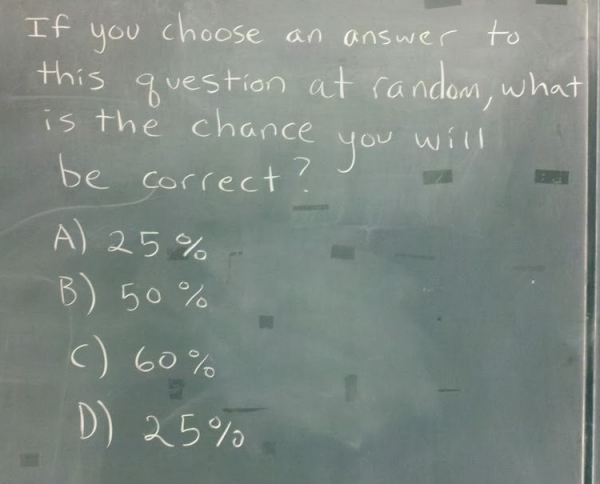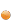## Friday, October 28, 2011Problem?

viaIf you liked this post, you can subscribe to the Blame It On The Voices RSS feed and get your regular fix

#### 13 comentarii:

Anonymous said...

B.

Anonymous said...

@Anon, No, because if you'd be right 50% of the time, then half of the answers must be 50%.

Anonymous said...

True

Pablo Nuñez said...

25%

Anonymous said...

B.

Anonymous said...

if two out of four answers are the same, then neither is correct.
so only B and C are possible.
so that's 50/50.
therefore, B.

Anonymous said...

Because 25% is listed twice, you have a 50% chance to be correct making B the right answer, therefore reducing the chance to be correct to 25% making A & D the right answers, therefore raising the chance to be correct to 50% and so and so... It is a paradox and the chance that you will be correct is 0% regardless the 4 choices and the percentage they show. After all, the question asks for a percentage as a final output, it doesn't limit you to the 4 choices as the only answers.

Anonymous said...

C

Anonymous said...

this is similar to the liar-paradox

Anonymous said...

What question? They don't ask you a question, so how can you determine the probability of being right? None of the answers is possible.

Anonymous said...

http://xkcd.com/169/

rishabh sharma said...

then
let me start from the beginning
we don't know which of these is the correct answer, so the probability of the answer being A (or D as they have same values) is 1/3 and the probability of us choosing A is 1/4 thus the probability of us being correct if answer is A is 1/3*1/4. similar applies for B,C and D. summing the probabilities we get (1/3*1/4)+(1/3*1/4)+(1/3*1/4)+(1/3*1/4= 1/3 or 33.33%.
now 33.33% is the correct solution to this problem, but if you read the question carefully you'll see it asks us to pick a choice out of the 4. now as 33.33% is not in the options we can never anser it correct, thus our probability being 0!
voila

Anonymous said...

What is the diversity of the book Knick knack patty whack